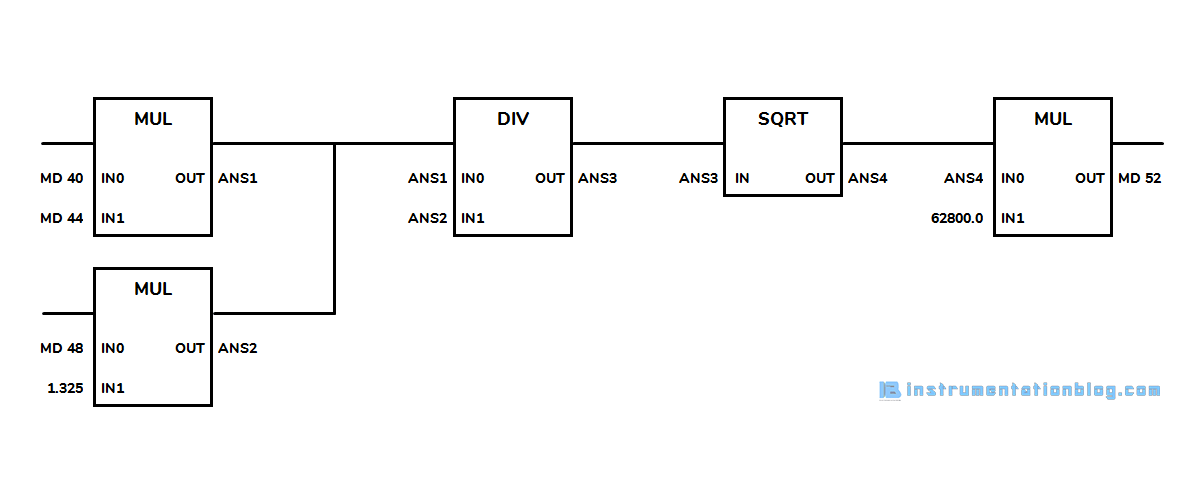# 20+ Arithmetic Instructions in PLC Programming | Free PLC Tutorials

While doing a program in plc, it is necessary to use arithmetic instructions in plc to calculate some specific functions like calculate flow rate, closed-loop control functions, PID Control functions, calculate power consumption, etc.

In my previous blog, we have seen the use of bit logic instructions in PLC programming, motor starter conversion in the plc program.

Programmable Logic Controller has arithmetic functions like Comparator, Mathematical, Logarithmic, etc.

In this blog, we are covering all these instructions like How to use these instructions? Application in Real-life conditions? etc.

So, Let’s see all these instructions one by one.

## Arithmetic Instructions in PLC Programming

The PLC arithmetic functions consist of many instructions like Comparison, Mathematical, Logarithmic, etc.

Out of all these instructions, we will first see the PLC Comparison instructions.

### List of PLC Comparison instructions:

• Equal: CMP ==
• Not Equal: CMP <>
• Greater Than: CMP >
• Less Than: CMP <
• Greater Than or Equal: CMP >=
• Less Than or Equal: CMP <=Equal: CMP == Tests whether two values are equal. If values are equal then this instruction is logically true.

Not Equal: CMP <> Tests whether two values are not equal. If values are not equal then this instruction is logically true.

Greater Than: CMP > Compares two values, If the value of IN0 is greater than the value of IN1 then this instruction is logically true.

Less Than: CMP < Compares two values, If the value of IN0 is less than the value of IN1 then this instruction is logically true.

Greater Than or Equal: CMP >= Compares two values, If the value of IN0 is greater than or equal to the value of IN1 then this instruction is logically true.

Less Than or Equal: CMP <= Compares two values, If the value of IN0 is less than or equal to the value of IN1 then this instruction is logically true.

Let’s take a simple example of giving an alarm indication to the operator when the temperature of the furnace reaches above 850-degree centigrade.In the above example, we are getting temperature value in the memory address MD50, we compare the value of MD50 with 850.0.

When the value reaches above 850.0 then it will activate the output Q0.0, which is directly connected with a hooter or indicator that will give an alarm signal to the operator.

### List of Arithmetic Instructions in PLC:

• Subtraction: SUB
• Multiplication: MUL
• Division: DIV
• Return Fraction: MOD
• Absolute: ABS
• Square Root: SQRT
• Square: SQR
• Exponential: EXP
• Natural Logarithm: LN
• Sine Value: SIN
• Cosine Value: COS
• Tangent Value: TAN
• Arc Sine Value: ASIN
• Arc Cosine Value: ACOS
• Arc Tangent Value: ATANAddition: ADD Adds the two values, IN0 with IN1, and stores the resulting value in the OUT.

Subtraction: SUB Subtracts the value of IN1 from IN0 and stores the resulting value in the OUT.

Multiplication: MUL Multiplies the two values, IN0 with IN1, and stores the resulting value in the OUT.

Division: DIV Divides the value of IN1 from IN0 and stores the resulting value in the OUT.

The input and output values must be Integer or Floating Point value in ADD, SUB, MUL, and DIV instructions.

Return Fraction: MOD Divides the value of IN1 from IN0 and stores the remainder value in the OUT.

The input and output values must be the Integer value in the MOD instruction.

Absolute: ABS Returns the absolute value of IN in the OUT.

Square Root: SQRT Returns the square root value of IN in the OUT.

Square: SQR Returns the square value of IN in the OUT.

Exponential: EXP Returns the exponential value of IN to the base e in the OUT.

Natural Logarithm: LN Returns the natural logarithm value of IN in the OUT.

Sine Value: SIN Returns the sine value of IN in the OUT.

Cosine Value: COS Returns the cosine value of IN in the OUT.

Tangent Value: TAN Returns the tangent value of IN in the OUT.

Arc Sine Value: ASIN Returns the arcsine value of IN in the OUT.

Arc Cosine Value: ACOS Returns the arc cosine value of IN in the OUT.

Arc Tangent Value: ATAN Returns the arctangent value of IN in the OUT.

The input and output values must be a Floating Point value in EXP, LN, SIN, COS, TAN, ASIN, ACOS, and ATAN instructions.

### Example of Flow Calculation

For a better understanding of these arithmetic instructions in plc programming, let’s take an example. Find the flow by the formula given below,In this formula we have d = pipe diameter, pi is a constant, ∆P is the differential pressure and P is the line pressure.

d = pipe diameter is fixed that is around 100mm, therefore we are getting 8π*(100/2)^2 = 62800.

We are getting ∆P in MD40, T in MD44, and P in MD48. Now, this is the ladder logic conversion of this formula.In the above figure, we are multiplying MD10(Differential Pressure) with MD14(Temperature) and saving its answer to ANS1.

Parallel to this, we do multiplication of MD18(Line Pressure) with 1.325 and saving its answer to ANS2.

Then divide ANS1 with ANS2 and saving its answer to ANS3 and do the square root of this value. Then in the final step multiply the answer of square root’s ANS4 to 62800. The resulting value will save into MD52.

These are the steps to find the Flow as per the formula is given above.

I hope you like this blog about the basic arithmetic instructions in PLC. Feel free to submit your feedback in the comment section below.

### 3 thoughts on “20+ Arithmetic Instructions in PLC Programming | Free PLC Tutorials”

1.Nice one. I have learnt a lot from your Site. Keep updating us the more.

2.•